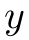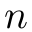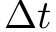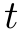Nuclear Pumpkin Mocha

# npm

## ode-rk4

1.1.3 • Public • Published

# ode-rk4Integrate a system of ODEs using the Fourth Order Runge-Kutta (RK-4) method

## Introduction

This module integrates a system of ordinary differential equations of the formwhereis a vector of length. Given time step, the Runge-Kutta 4 method integrates the ODE with updatewhereare given byFor a similar adaptive method using the fifth order Cash-Karp Runge-Kutta method with fourth order embedded error estimator, see [https://github.com/scijs/ode45-cash-karp](ode45-cash-karp].

## API

### require('ode-rk4')( y0, deriv, t0, dt )

Arguments:

• y0: an array or typed array containing initial conditions. This vector is updated in-place with each integrator step.
• deriv: a function that calculates the derivative. Format is function( dydt, y, t ). Inputs are current state y and current time t, output is the calculated derivative dydt.
• t0: initial time.
• dt: time step.

Returns: Initialized integrator object.

Properties:

• n: dimension of y0.
• y: current state. Initialized as a shallow copy of input y0.
• deriv: function that calculates the derivative. Initialized from input. May be changed.
• t: current time, incremented by dt with each time step.
• dt: time step. Initialized from input dt. May be changed.

Methods:

• .step(): takes a single step of the RK-4 integrator and stores the result in-place in the y property.
• .steps( n ): takes n steps of the RK-4 integrator, storing the result in-place in the y property.

## Credits

(c) 2015 Ricky Reusser. MIT License

## Keywords

### Install

npm i ode-rk4

### Repository

github.com/scijs/ode-rk4